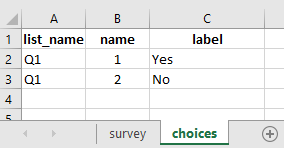# Assigning a certain value from a question's choice (composite index)

From select question I want pass value like 1,3,4

Hi @mahabbat
Welcome to the community forum. I would suggest that you provide more details on what exactly you were trying to do and what challenges are you experiencing.

Stephane

1 Like

Question: Do you Saving?
If yes then you will get = 210= 20 BDT (yes =2)
If No then You will get = 1
5= 5 BDT (No=1)

How I will do it by selective question?

Hi @mahabbat,

Correct me if i got you correctly:

You have a question …

Q1. Are you saving?

It’s choices are as follows:

1. No
2. Yes

So, it seems that you are trying to give a value of 20 BDT (i.e. 210) if `yes` and 5 BDT (i.e. 15) if `no`. Is this what you are trying to do?

If select Yes then Yes pass the value Yes =2 value and further I want multiply with Integer value

So what is the multiplication that you wish to see …

For yes (choice coded as 2) … and for no (choice coded as 1).

Choice

1. Yes
2 No

if select Yes your mark is 4 and total mark is 4 multiply with 10 = 40
If select No You mark is 2 and total mark is 2 multiply with 5 = 10

how I make from ? help me

OK, you could do the same as outlined in the image shared below:

### In the choices tab of your xlsform:### Reference xlsform:

Weighted Score Sample.xlsx (11.4 KB)

1 Like

Thank you very much, Your support is much appreciated and we have done it by your help

2 Likes